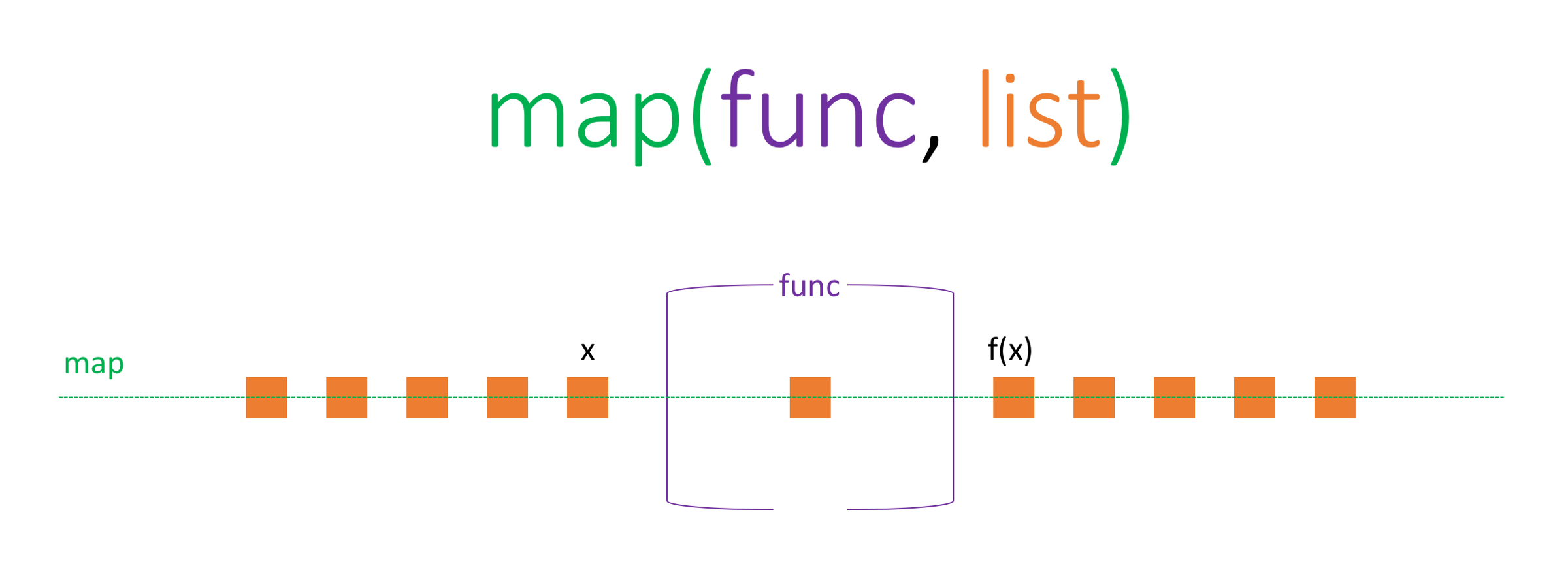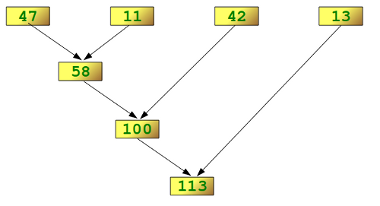# Functional programming with python 

December 14, 2017

### Functional Programming with Python

As very well explained by David Mertz, a director of Python Software Foundation:

Python is not a functional programming language, but it is a multi-paradigm language that makes functional programming easy to perform, and easy to mix with other programming styles.

Main goal of this post is to have a quick look on some functional programming tools in Python, which are lambda reduce andmap . I will try to solve some simple tasks with functional and non-functional style. You can find a few more examples on the github repository.

### 1. lambda

Python supports the creation of anonymous functions (i.e. functions that are not bound to a name) at runtime, using a construct called "lambda". This is not exactly the same as lambda in functional programming languages, but it is a very powerful concept that's well integrated into Python and is often used in conjunction with typical functional concepts like `filter()`, `map()` and `reduce()`.
If you are familiar with ECMA6, `lambda` has similar syntax with arrow => function.
Syntax: `lambda input: output`
You can use the given input, on `:`right side of colon.
Lets start coding with a function called `reverseString`, which simply returns the reverse of given string. You can write the code in regular function definition as:

``````def reverseString(string):
return string[::-1]``````

And we can write the same function using lambda as follows:

``reverseString = lambda string: string[::-1]``

Greet given name as `Hello \${name}`

``````# regular definition
def greet(name):
return 'Hello ' + name

# lambda definition
greet = lambda name: 'Hello ' + name``````

`lambda` in Python comes with limitations, consider followings when using it:

• does not accept multiple definitions

• you can not make assignment on lambda function's body.

### 2. map( )

Map function, iterates through given iterable and applies the given function on each individual elements.

Syntax: `map(function, iterable_list)`

``````# If you are using Python 2.x, import print_function
# from __future__ import print_function

primaryColors = ['red', 'green', 'blue']

# regular definition
for color in primaryColors:
print(color)

# map definition
map(print, primaryColors)``````

Cool, isn't it?map returns an 'iterator' object in Python, as explained in Python docs:

object representing a stream of data. Repeated calls to the iterator's __next__() method (or passing it to the built-in function next()) return successive items in the stream.

I am not going to deep dive into definitions and limitations on this post, let's get back to coding. To combine what we've learned so far, let's greet a bunch of people.

``````people = ['Mahmut', 'Elliot', 'Lee', 'Brady', 'Tanya']

def greet(name):
return 'Hello ' + name
for person in people:
print(greet(person))

greet = lambda person: 'Hello ' + person
map(print, map(greet, people))``````

As explained in Python docs, we can use result of map function - which is `map(greet, people)` part- as iterable.
Also because map takes `function` as first input and lambda actually is a function, we can use lambda inside map definition.

``map(lambda person: print('Hello ' + person), people)``

Finally, we have a pure functional definition doing stuffs in one line of code. Let's check reduce function next.

### 3. reduce( )

If you are familiar with ECMA6 syntax, reduce in Python has quite similar syntax and functionality.
Syntax: `reduce(function, sequence, *[initial_value])`

The function reduce(func, seq) continually applies the function func() to the sequence seq. It returns a single value as explained in this document.

Let's check very common example shared in the doc.

``reduce(lambda x, y: x + y, [47, 11, 42, 13])``reduce(func, seq)

Returns the sum of numbers array, which is an integer value, instead array of numbers. So what reduce does is simply;

• get the first element of iterable or initial value → seq

• apply given function on it → func(seq)

• initialize output section with result of func → output = func(seq)

• get second element of iterable → seq

• execute given multiple parameters function on it → func(seq, output)

and so on…

For example, we can code mean calculation function as follows:

``````# with regular definition
def calculate_mean(list):
total = 0
for number in list:
total += number